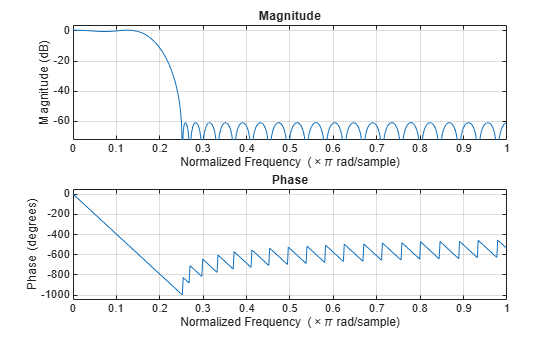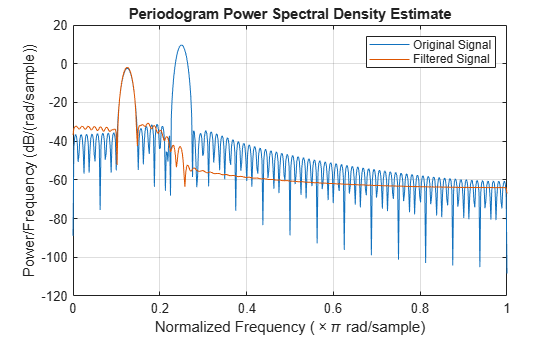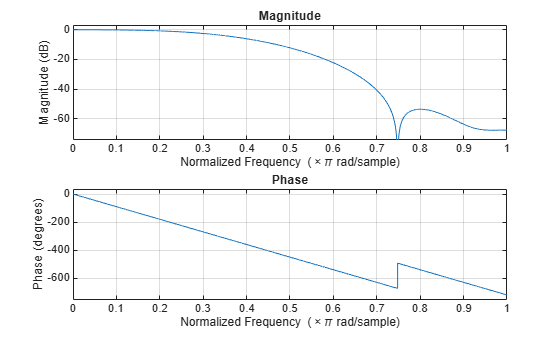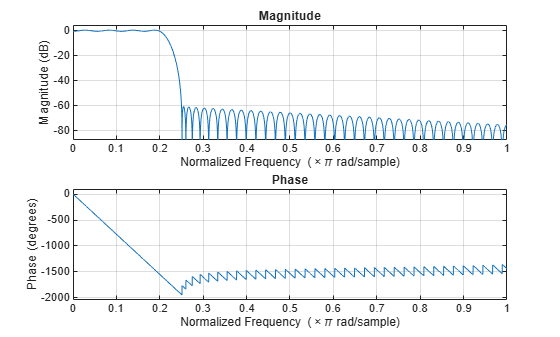Documentation

This is machine translation

Mouseover text to see original. Click the button below to return to the English version of the page.

fdesign.lowpass

Lowpass filter specification

Syntax

D = fdesign.lowpass D = fdesign.lowpass(SPEC) D = fdesign.lowpass(SPEC,specvalue1,specvalue2,...) D = fdesign.lowpass(specvalue1,specvalue2,specvalue3,specvalue4) D = fdesign.lowpass(...,Fs) D = fdesign.lowpass(...,MAGUNITS) 

Description

D = fdesign.lowpass constructs a lowpass filter specification object D, applying default values for the default specification option 'Fp,Fst,Ap,Ast'.

D = fdesign.lowpass(SPEC) constructs object D and sets the Specification property to the entry in SPEC. Entries in SPEC represent various filter response features, such as the filter order, that govern the filter design. Valid entries for SPEC are shown below. The options are not case sensitive.

Note

Specifications options marked with an asterisk require the DSP System Toolbox™ software.

• 'Fp,Fst,Ap,Ast' (default option)

• 'N,F3db'

• 'N,F3db,Ap' *

• 'N,F3db,Ap,Ast' *

• 'N,F3db,Ast' *

• 'N,F3db,Fst' *

• 'N,Fc'

• 'N,Fc,Ap,Ast'

• 'N,Fp,Ap'

• 'N,Fp,Ap,Ast'

• 'N,Fp,Fst,Ap' *

• 'N,Fp,F3db' *

• 'N,Fp,Fst'

• 'N,Fp,Fst,Ast' *

• 'N,Fst,Ap,Ast' *

• 'N,Fst,Ast'

• 'Nb,Na,Fp,Fst' *

The filter specifications are defined as follows:

• Ap — amount of ripple allowed in the pass band in decibels (the default units). Also called Apass.

• Ast — attenuation in the stop band in decibels (the default units). Also called Astop.

• F3db — cutoff frequency for the point 3 dB point below the passband value. Specified in normalized frequency units.

• Fc — cutoff frequency for the point 6 dB point below the passband value. Specified in normalized frequency units.

• Fp — frequency at the start of the pass band. Specified in normalized frequency units. Also called Fpass.

• Fst — frequency at the end of the stop band. Specified in normalized frequency units. Also called Fstop.

• N — filter order.

• Na and Nb are the order of the denominator and numerator.

Graphically, the filter specifications look similar to those shown in the following figure.Regions between specification values like Fp and Fst are transition regions where the filter response is not explicitly defined.

D = fdesign.lowpass(SPEC,specvalue1,specvalue2,...) constructs an object D and sets the specification values at construction time using specvalue1, specvalue2, and so on for all of the specification variables in SPEC.

D = fdesign.lowpass(specvalue1,specvalue2,specvalue3,specvalue4) constructs an object D with values for the default Specification property 'Fp,Fst,Ap,Ast' using the specifications you provide as input arguments specvalue1,specvalue2,specvalue3,specvalue4.

D = fdesign.lowpass(...,Fs) adds the argument Fs, specified in Hz to define the sampling frequency to use. In this case, all frequencies in the specifications are in Hz as well.

D = fdesign.lowpass(...,MAGUNITS) specifies the units for any magnitude specification you provide in the input arguments. MAGUNITS can be one of

• 'linear' — specify the magnitude in linear units

• 'dB' — specify the magnitude in dB (decibels)

• 'squared' — specify the magnitude in power units

When you omit the MAGNUNITS argument, fdesign assumes that all magnitudes are in decibels. Note that fdesign stores all magnitude specifications in decibels (converting to decibels when necessary) regardless of how you specify the magnitudes.

Examples

collapse all

Lowpass filter a discrete-time signal consisting of two sine waves.

Create a lowpass filter specification object. Specify the passband frequency to be $0.15\pi$ rad/sample and the stopband frequency to be $0.25\pi$ rad/sample. Specify 1 dB of allowable passband ripple and a stopband attenuation of 60 dB.

d = fdesign.lowpass('Fp,Fst,Ap,Ast',0.15,0.25,1,60);

Query the valid design methods for your filter specification object, d.

designmethods(d)
Design Methods for class fdesign.lowpass (Fp,Fst,Ap,Ast): butter cheby1 cheby2 ellip equiripple ifir kaiserwin multistage 

Create an FIR equiripple filter and view the filter magnitude response with fvtool.

Hd = design(d,'equiripple'); fvtool(Hd)Create a signal consisting of the sum of two discrete-time sinusoids with frequencies of $\pi /8$ and $\pi /4$ rad/sample and amplitudes of 1 and 0.25, respectively. Filter the discrete-time signal with the FIR equiripple filter object, Hd.

n = 0:159; x = (0.25*cos((pi/8)*n)+sin((pi/4)*n)); y = filter(Hd,x);

Compute the Fourier transform of the original signal and the filtered signal. Verify that the high-frequency component has been filtered out.

freq = 0:(2*pi)/160:pi; xdft = fft(x); ydft = fft(y); figure plot(freq/pi,abs(xdft(1:length(x)/2+1))) hold on plot(freq/pi,abs(ydft(1:length(y)/2+1))) hold off legend('Original Signal','Filtered Signal') ylabel('Magnitude') xlabel('Normalized Frequency (\times\pi rad/sample)')Create a filter of order 10 with a 6-dB frequency of 9.6 kHz and a sampling frequency of 48 kHz. Look at the available design methods.

d = fdesign.lowpass('N,Fc',10,9600,48000); designmethods(d)
Design Methods for class fdesign.lowpass (N,Fc): window 

The only valid design method is the FIR window method. Design the filter.

Hd = design(d);

Display the filter magnitude response. The -6 dB point is at 9.6 kHz, as expected.

fvtool(Hd)Create an FIR equiripple filter with a passband frequency of $0.2\pi$ rad/sample, a stopband frequency of $0.25\pi$ rad/sample, a passband ripple of 1 dB, and a stopband attenuation of 60 dB. Design the filter with a 20 dB/rad/sample linear stopband.

D = fdesign.lowpass('Fp,Fst,Ap,Ast',0.2,0.25,1,60); Hd = design(D,'equiripple','StopbandShape','linear','StopbandDecay',20);

Visualize the frequency response of the filter.

fvtool(Hd)